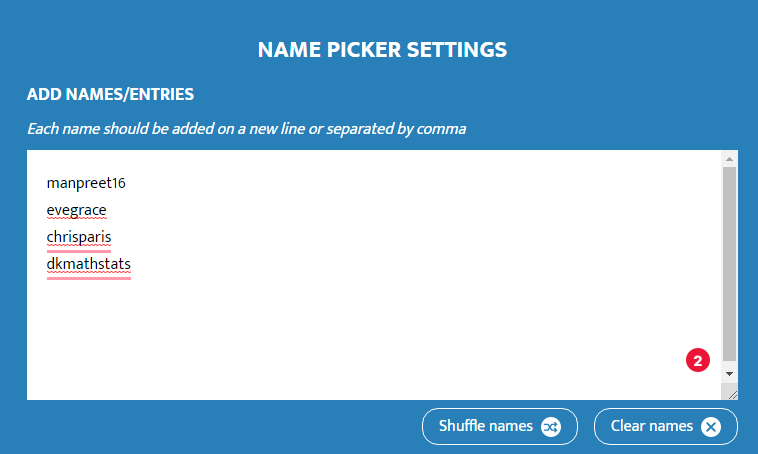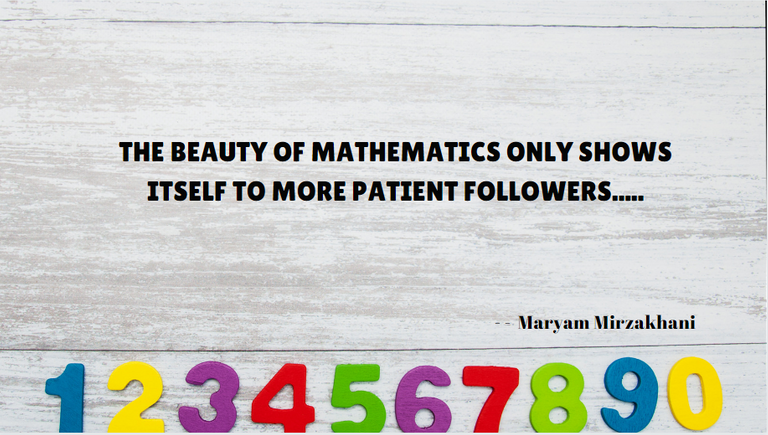# Maths Brain Teasers 42:: Eliminate the ODD One Out???

in STEMGeeks2 months ago

Hey All; @StemGeeks Mathematician;Last time when we had the ODD one puzzle to solve it was FUN. We had a lot of entries to the contents and at the same time, folks asked for similar puzzles. Considering their request, our Maths Brain-teaser for today is around;

### Find The ODD One Out???

Have a close look at the image given and see if you find the ODD one out. There is a specific reason to eliminate the ODD number and that can only be done via logic.. Hence; see that you find/get to the logic to element the number which is ODD. I cannot give a HINT to this puzzle coz. if I give the hint you should be able to solve it very easily.. therefore no HINT for todays' puzzle. With that, I'll leave you all with the Maths Brain Teaser for today:: Find The ODD One Out??? Good luck solving iT...

##### Maths Brain Teasers 41:: Can You Find the Missing Number?- Solved with ExplanationFollowing is the logic to solve the Maths Brain Teasers 41:: Can You Find the Missing Number??

• Step 1 The first row Number is Divided by the Second Row First Number; for example

### 70 / 14 = 5;

• Step 2 In a Similar fashion we take the First Row second column number to be dived by the second row; second column Number i.e.

### 91 / 13 = 7

Finally the Row in Question;

The third Row Number; Third column Number is divided by; Secon row Third Column Number i.e.;

### 120 / 24 = 5

Hence the Answer to:: Maths Brain Teasers 41:: is 5

### STEM token GiveAway

I'll be again doing a giveaway of STEM tokens to the lucky random winner with the correct answer. For the last contest, which was Maths Brain Teasers 41:: Can You Find the Missing Number??.

We had close to 4 entries and all of them provided the correct answers to the Maths puzzle. I'm also surprised to look at the detailed answers provided by everyone as to how they arrived at the solution of the puzzle.. Well done guys.. Way to GO...

All the entries provided the right answer. Hence; I'm going to use the comment picker tool to select a random winner for this Maths Brain Teasers 41 - Can You Find the Missing Number??Congratulations @chrisparis; You WIN yourself 10 STEM tokens. You should be having the rewards in your STEM Wallet Soon.### Math Quote for the Day::

Here is the motivation to solve the Maths Equation Puzzle?If you like my work, then please spread the Word.. that we do have the Math Brain Teasers competition here @StemGeeks platform. Reblog is much appreciated.

Best RegardsFind Me on the Other Social Media Platforms::PS:: All the Maths Brain Teasers; are made by me using the Pro Canva License Version

Sort:

Cool I'm lucky!!!
4 x4 = 16
4x5 = 20
4x7 = 28
4x8 = 32
4x9 = 36
4x14 = 56
18 alone...
Answer maybe 18

2 months ago (edited)

Looks like its getting exciting - I could never solve any when I tried to look - you seem to choose the complex ones or my brain has lost the power to solve these 😀

5 ? That looks odd to me - no multiples of it present.

18 is odd one out as others are factors of 4 but no factor of 18 with 4 is there.

18?